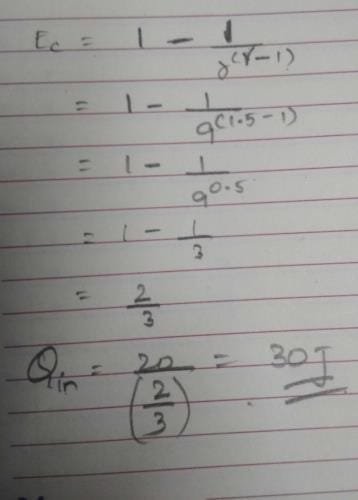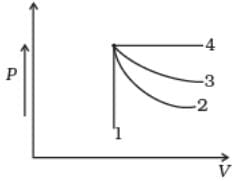NEET  >  Test: Heat Engines

# Test: Heat Engines

Test Description

## 10 Questions MCQ Test Physics Class 11 | Test: Heat Engines

Test: Heat Engines for NEET 2023 is part of Physics Class 11 preparation. The Test: Heat Engines questions and answers have been prepared according to the NEET exam syllabus.The Test: Heat Engines MCQs are made for NEET 2023 Exam. Find important definitions, questions, notes, meanings, examples, exercises, MCQs and online tests for Test: Heat Engines below.
Solutions of Test: Heat Engines questions in English are available as part of our Physics Class 11 for NEET & Test: Heat Engines solutions in Hindi for Physics Class 11 course. Download more important topics, notes, lectures and mock test series for NEET Exam by signing up for free. Attempt Test: Heat Engines | 10 questions in 10 minutes | Mock test for NEET preparation | Free important questions MCQ to study Physics Class 11 for NEET Exam | Download free PDF with solutions
 1 Crore+ students have signed up on EduRev. Have you?
Test: Heat Engines - Question 1

### In a heat engine the ratio of net work done per cycle by the engine to the total amount of heat absorbed per cycle by the working substance from the source is known as

Detailed Solution for Test: Heat Engines - Question 1

In a heat engine the ratio of net work done per cycle by the engine to the total amount of heat absorbed per cycle by the working substance from the source is known as Thermal Efficiency.

Test: Heat Engines - Question 2

### In actual home refrigerator vapours of Freon ( which is dichlorodifluoro methane CCl2F2) act as

Detailed Solution for Test: Heat Engines - Question 2

Dichlorodifluoromethane (R-12) is a colorless gas usually sold under the brand name Freon-12, and a chlorofluorocarbon halomethane (CFC) used as a refrigerant and aerosol spray propellant. Complying with the Montreal Protocol, its manufacture was banned in developed countries (non-article 5 countries) in 1996, and developing countries (article 5 countries) in 2010 due to concerns about its damaging impact to the ozone layer.[better source needed] Its only allowed usage is as fire retardant in submarines and aircraft. It is soluble in many organic solvents. Dichlorodifluoromethane was one of the original propellants for Silly String. R-12 cylinders are colored white.

Test: Heat Engines - Question 3

### A carnot engine is taking 700 cal from source and is rejecting 500 cal to the sink in each cycle. What is the temperature of sink if the source temperature is 150° C .

Detailed Solution for Test: Heat Engines - Question 3

Heat extracted from source Q1= 700 cal
Heat rejected to the sink Q2= 500 cal
Temperature of source T1= 150 +273= 423K
Temperature of sink T2 to be found:
Q2/Q1 = T2/T1
So,
T2 = (Q2/Q1) x T1
= (500 x 423)/700
= 302.02 K
= 29.02 degree C.

Test: Heat Engines - Question 4

According to Carnot theorem no heat engine working between two given temperatures of source and sink can be more efficient than a perfectly ___________ engine working between the same two temperatures

Detailed Solution for Test: Heat Engines - Question 4

According to Carnot theorem no heat engine working between two given temperatures of source and sink can be more efficient than a perfectly reversible engine working between the same two temperatures, by the statement of Carnot theorem.

Test: Heat Engines - Question 5

How much heat energy a petrol engine would require to do 20 kWh of work? Its adiabatic compression ratio is 9 and γ= 1.5.

Detailed Solution for Test: Heat Engines - Question 5Test: Heat Engines - Question 6

An ideal gas undergoes four different processes from the same initial state (Fig.). Four processes are adiabatic, isothermal, isobaric and isochoric. Out of 1, 2, 3 and 4 which one is adiabatic.Detailed Solution for Test: Heat Engines - Question 6

4 is isobaric process, 1 is isochoric. Out of 3 and 2, 3 has the smaller slope (magnitude) hence is isothermal. Remaining process 2 is adiabatic.

Test: Heat Engines - Question 7

What would be the horse power of a steam engine with average pressure of steam 9x 104 Nm-2 , the area of cross section of the piston is 0.2 m2, length of stroke is 0.6 m and piston makes 5 revolutions per second?

Detailed Solution for Test: Heat Engines - Question 7

Energy in 1 revolution=2 x P x a x l=2 x 9 x104 x 0.2 x0.6 j=21600J
Energy in n revolutions= 21600nJ
Power=E/t=21600n/t=21600 x 5=18000 J/s= 108000/746 h.p=144.77 h.p.

Test: Heat Engines - Question 8

The ratio of quantity of heat removed per cycle from the contents of the refrigerator to the energy spent per cycle to remove this heat is called the

Detailed Solution for Test: Heat Engines - Question 8

The ratio of quantity of heat removed per cycle from the contents of the refrigerator to the energy spent per cycle to remove this heat is called the coefficient of performance. It is the definition of coefficient of performance.

Test: Heat Engines - Question 9

In a Carnot engine 800 J of heat is absorbed from a source at 400 K and 640 J of heat is rejected to the sink. The temperature of the sink is:

Detailed Solution for Test: Heat Engines - Question 9

for hot reservoir I.e. source
temperature T1=400k
heat supplied Q1=800J
For cold reservoir i.e. sink
Temperature T2=?
Heat supplied Q2=640J
According to carnot’s theorem,
Q1/Q2= T2/T1
640/800= T2/400
T2=320k

Test: Heat Engines - Question 10

The temperature inside a refrigerator is 4°C and the room temperature is 27°C. How many joules of heat will be delivered to the room for each joule of electricity consumed by the refrigerator?( Treat the refrigerator as ideal).

Detailed Solution for Test: Heat Engines - Question 10

Coefficient of performance(cop) of a refrigerator,
β= Q2/Q1-Q2=T2/T1-T2=277/300-277=277/23≈12
OR, Q2/W=12. Therefore, W=1J.Q2=12J
Also, Q1=Q2+W
=(12+1) J
=13J

## Physics Class 11

127 videos|464 docs|210 tests
 Use Code STAYHOME200 and get INR 200 additional OFF Use Coupon Code
Information about Test: Heat Engines Page
In this test you can find the Exam questions for Test: Heat Engines solved & explained in the simplest way possible. Besides giving Questions and answers for Test: Heat Engines, EduRev gives you an ample number of Online tests for practice

## Physics Class 11

127 videos|464 docs|210 tests

### How to Prepare for NEET

Read our guide to prepare for NEET which is created by Toppers & the best Teachers